# An exponential sum involving primes

Suppose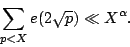If that bound held for some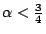then one could extend the current results on the 1-level density of low-lying zeros of cusp form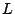-functions. This would imply (assuming GRH for the critical zeros of these-functions) that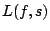has no Siegel zeros in a strong sense. Namely, that there exists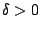such thatis nonzero for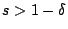.

(References and more details on the above are sought).

It is tempting to think that the above estimate must surely hold for any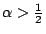, because one naturally expects square-root cancellation in any random looking'' sum. However, the following example shows that nature is more subtle than that. Let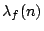denote the (suitably normalized) Fourier coefficients of a holomorphic cusp form. Then [Conrey and Ghosh?, unpublished?] have shown that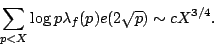The lack of cancellation in that sum is puzzling.

Back to the main index for L-functions and Random Matrix Theory.